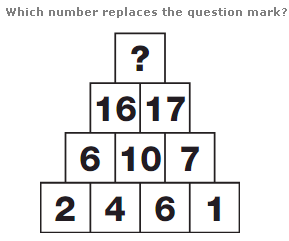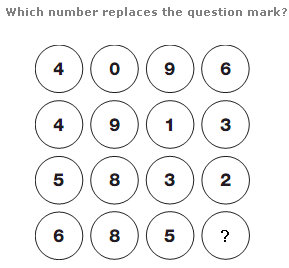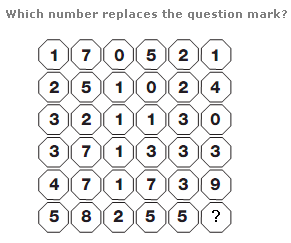# Puzzles - Number puzzles

### Exercise :: Number puzzlesAnswer : 33 Explanation : Starting with numbers on the bottom row, add numbers in adjacent boxes together, and put the result in the box directly above. Continue in this way, up the triangle.Answer : 9 Explanation : Reading each row as a 4 digit number, from top to bottom, rows follow the sequence of Cube Numbers from 16 to 19.Answer : 1 Explanation : Split the diagram into 3 columns, each 2 spaces wide, and read each double space as a 2 digit number. Starting at the top of each column, add the 2 separate digits together, then add this to the whole 2 digit number, to give the result, written in the spaces underneath.